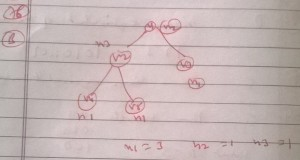# GATE | Gate IT 2008 | Question 73

A binary tree with n > 1 nodes has n1, n2 and n3 nodes of degree one, two and three respectively. The degree of a node is defined as the number of its neighbors.

n3 can be expressed as
(A) n1 + n2 – 1
(B) n1 – 2
(C) [((n1 + n2)/2)]
(D) n2 – 1

Explanation: